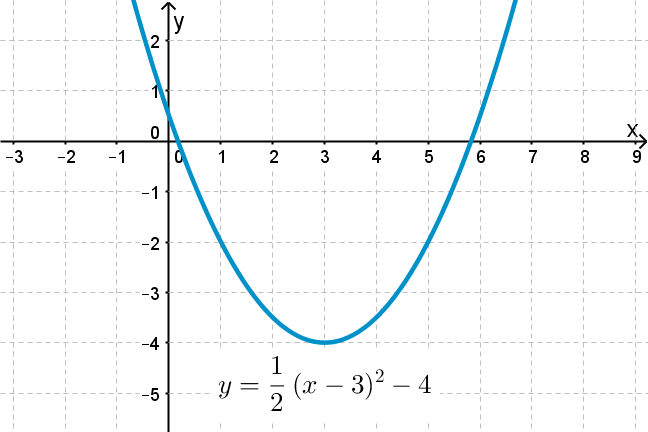## Want to keep learning?

This content is taken from the UNSW Sydney's online course, Maths for Humans: Linear, Quadratic & Inverse Relations. Join the course to learn more.
2.8

## UNSW SydneyA parabola translated from the origin

# Scaling and translating quadratic functions

We can get more general quadratic functions by scaling and translating the standard equation $$\normalsize{y=x^2}$$ . Pleasantly, any quadratic function can be obtained in this way.

In this step we will see how scaling and translation affect the graph of a quadratic function.

## Scaling the standard parabola

We have already seen that the shape of $$\normalsize{y=ax^2}$$ depends on the value of the constant $$\normalsize{a}$$. We can consider this graph to be a scaling of the particular standard parabola $$\normalsize{y=x^2}$$; if $$\normalsize{a>0}$$ then the parabola opens upwards, and if $$\normalsize{a<0}$$ then the parabola opens downwards.

For large values of $$\normalsize{a}$$ the parabola is steep and narrow. The closer to $$\normalsize{0}$$ the value $$\normalsize{a}$$ gets, the flatter the parabola. Indeed when $$\normalsize{a=0}$$ the parabola is so flat that we get the straight line conic $$y=0$$, which could be considered as a special case of the parabola.

Here for comparison are the graphs of $$\normalsize{y=4x^2}$$ (green), $$\normalsize{y=x^2}$$ (blue) and $$\normalsize{y=\frac{x^2}{4}}$$ (red).## Translating a standard parabola up or down

Now we investigate what happens if we translate a standard parabola $$\normalsize{y=ax^2}$$ up or down. This is quite easy. Note that however we translate a parabola, its vertex, and focus, and directrix will move in exactly the same way.

Q1 (E): Where is the vertex of the parabola obtained by translating $$\normalsize{y=4x^2}$$ by $$\normalsize{3}$$ in the $$\normalsize{x}$$ direction and by $$\normalsize{5}$$ in the $$\normalsize{y}$$ direction?

Geometrically, if we add a constant $$\normalsize{k}$$ to the equation $$\normalsize{y=ax^2}$$ then we translate the parabola by $$\normalsize{k}$$ in the vertical direction. The algebraic operation that shifts a function vertically by $$k$$ can be thought of as replacing $$y$$ with $$y-k$$.

Recall that the parabola $$\normalsize y=\frac{1}{4} x^2$$ has vertex $$\normalsize V=[0,0]$$, focus $$\normalsize F=[0,1]$$, and directrix $$\normalsize l: y=-1$$. Here is a graph of the parabola $$\normalsize{y=\frac{1}{4}x^2-3}$$, obtained by translating $$\normalsize{y=\frac{1}{4}x^2}$$ down by $$\normalsize{3}$$. So its vertex will be at $$\normalsize{[0,-3]}$$, its focus will be at $$\normalsize{[0,-2]}$$, and its directrix will be the line $$\normalsize{ y=-4}$$.## Translating a standard parabola left or right

What if we want to shift $$\normalsize{y=\frac{1}{4}x^2}$$ over $$\normalsize{5}$$ units to the right? We apply the same strategy as when we were translating lines: replace $$x$$ with $$x-5$$ to get

$\Large{y=\frac{1}{4}(x-5)^2.}$

It is easy to check the old vertex $$\normalsize{[0,0]}$$ has moved to $$\normalsize{[5,0]}$$.We could expand the equation to get

$\Large{y=\frac{1}{4}(x-5)^2=\frac{1}{4}(x^2-10x+25)=\frac{1}{4}x^2-\frac{5}{2}x+\frac{25}{4}.}$

## Combining translations horizontal and vertical

If we take the standard parabola $$\normalsize{y=ax^2}$$ and translate it by $$\normalsize{h}$$ in the $$\normalsize{x}$$ direction and by $$\normalsize{k}$$ in the $$\normalsize{y}$$ direction, then we obtain

$\Large{y=a(x-h)^2+k}.$

This is the general form of a standard parabola which has been translated by the vector, or directed line segment, $$\normalsize{(h,k)}$$. This an important expression in the theory of quadratic functions.

Q2 (M): What is the vertex of this parabola?

Q3 (M): What are the focus and directrix of $$\normalsize{y=1/4(x-5)^2-2}$$?

Q4 (C): What is the focus of the parabola $$\normalsize{y=ax^2}$$?

## A key fact about parabolas

Can we get every parabola this way – just by taking a standard parabola of the form $$\normalsize{y=ax^2}$$, and then shifting or translating in the $$\normalsize{x}$$-direction by a certain amount and then in the $$\normalsize{y}$$-direction by a certain amount? Yes we can!

This is a pleasant and important fact about parabolas. It shows us that all quadratic functions, even ones with complicated formulas like $$\normalsize{y=7x^2-11x+8}$$ have essentially similar shapes, and that we can find out where they are situated by unravelling how much $$\normalsize{x}$$ and $$\normalsize{y}$$ translations are required to obtain them from standard parabolas.

So there is a fundamental question here: how can we translate a standard parabola to get the general parabola $$\normalsize{y=ax^2+bx+c}$$? Finding the answer will take us to ancient Persia, to a technique called completing the square, and to a remarkable identity that all students of mathematics ought to have seen.

A1. If we translate a parabola, then its vertex translates correspondingly. Since the vertex of $$\normalsize{y=4x^2}$$ is the origin $$\normalsize{[0,0]}$$, the vertex of the translated parabola must be $$\normalsize{[3,5]}$$.
A2. The unshifted parabola has a vertex at $$\normalsize{[0,0]}$$, so the new vertex will be at $$\normalsize{[h,k]}$$.
A3. The unshifted parabola $$y=\frac14 x^2$$ has focus at $$[0,1]$$ and directrix $$y=-1$$. We have essentially just moved the entire picture to the right by $$5$$ and down by $$2$$. So visually we can see the focus is now located at $$[5,-1]$$ and the directrix is now $$y=-3$$.
A4. The focus of the parabola $$\normalsize y=ax^2$$ is the point $$\normalsize F=[0,\frac{1}{4a}]$$.